# Normal Data

A number of statistical tools require that the underlying data be normally distributed.  Keep in mind that no real-world data-set is perfectly normal, but data should be checked to ensure that it is reasonably normal, when a given statistical tool requires it.  Note: The 3.4 DPM level associated with Six Sigma processes assumes normal data.

## First Option – Plot a Histogram

In the DMAIC world, plotting a histogram and looking at its shape is usually sufficient for checking normality.  The only exception is when sample sizes are very small, in which case a normal probability plot (below) may be the best approach.  Normally distributed data will form a bell-shaped histogram, with the highest bars in the middle, and progressively smaller bars toward the edges, as shown in the following data (randomly generated using MINITAB) –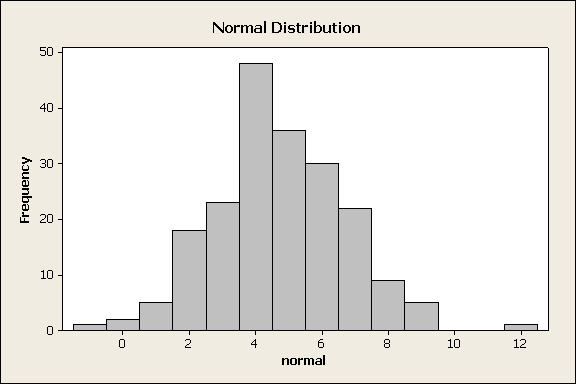## Second Option – Normal Probability Plot

Normal probability plots can take different forms, but all have one thing in common:  the closer the data points are to the theoretical-normal line, the more likely it is that the data is normal.

The normal probability plots below show data values along the x-axis, versus the cumulative percentage of data points collected, on the y-axis.  The blue line on the chart reflects a perfectly normal distribution: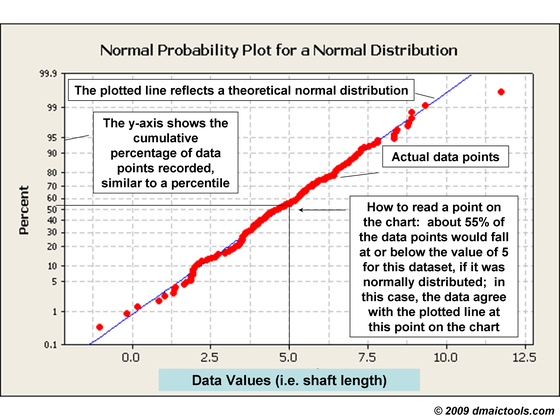Here are some examples of normal and non-normal data (made into histograms), and their corresponding probability plots (generated with MINITAB software).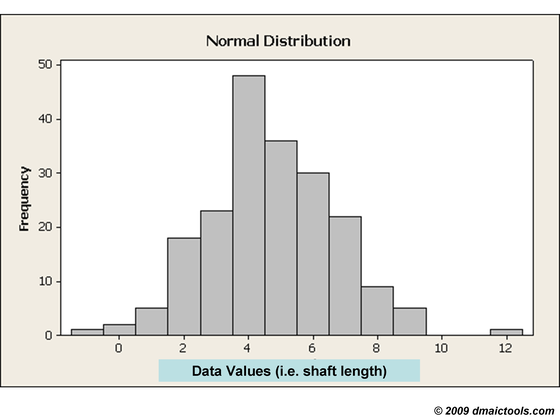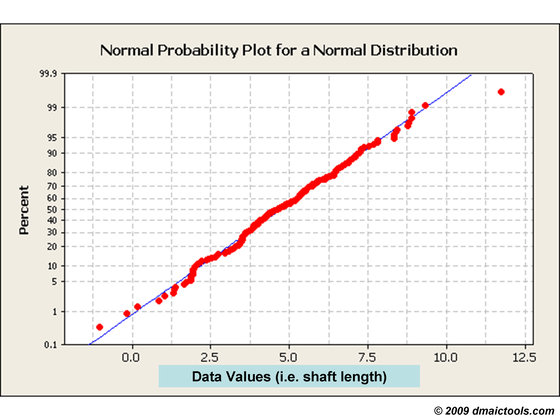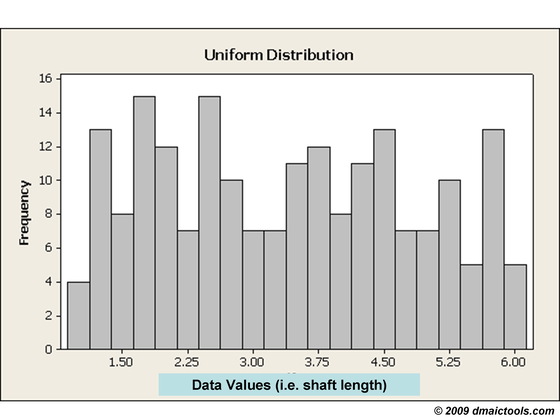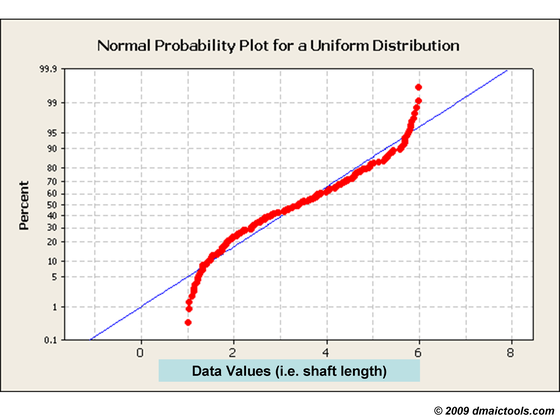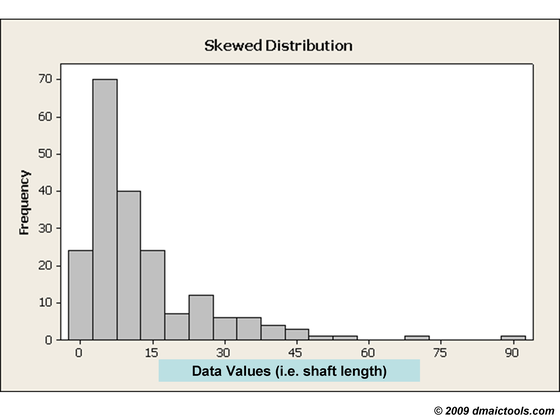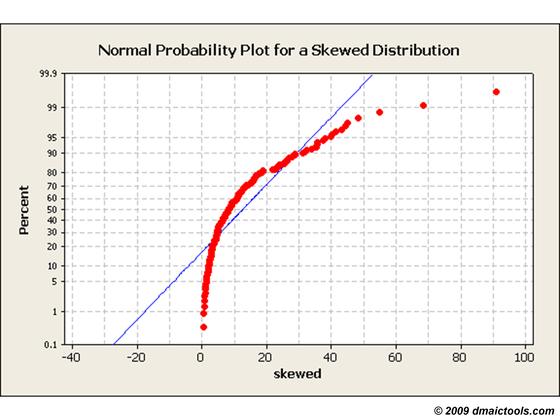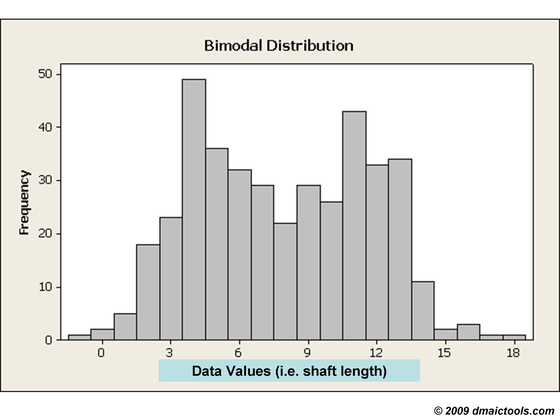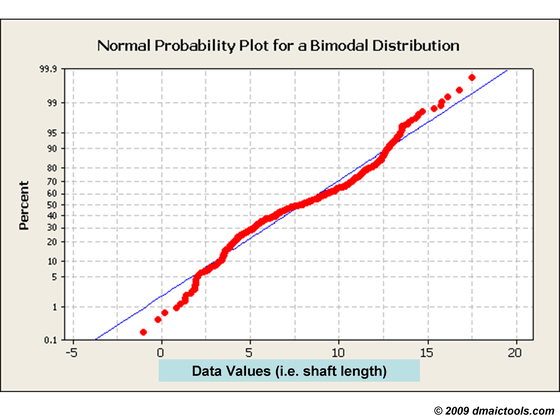Note that the histograms are as indicative of normality (or non-normality) as the probability plots in these cases.

Defect-Rate Predictions and Non-Normal Data

Statistical techniques are available for dealing with non-normal data, but we’d like to bring some “real-world” perspective into the discussion from a Six Sigma practitioner’s viewpoint – Six Sigma practitioners get paid to reduce variation, not to model variation.  It is far better for a team to put its energy into learning the underlying causes of variation than to get wrapped up in finding the correct distribution or transformation method to make defect-rate predictions.

Once the underlying causes are understood, process redesign and process control are much greater assurances of zero defects over the long run than the fact that a sample taken from the population happened to be normal and capable at one point in time.

So the message here is, there are very few cases where non-normal data should stop a project from moving forward.